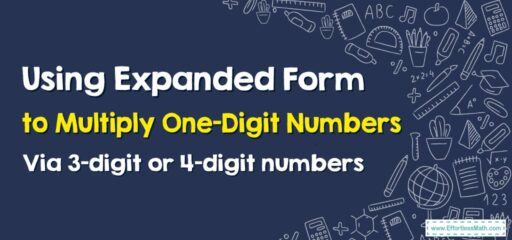# How to Use Expanded Form to Multiply One-Digit Numbers By 3-digit or 4-digit Numbers

Expanded form is a great way to simplify multiplication problems, especially when you're multiplying one-digit numbers with larger numbers.## A Step-by-step Guide to Using Expanded Form to Multiply One-Digit Numbers By 3-digit or 4-digit Numbers

Here is a step-by-step guide to Using Expanded Form to Multiply One-Digit Numbers Via 3-digit or 4-digit Numbers:

Example: Multiply 3 by 456 (a 3-digit number)

### Step 1: Understand the problem

You are given the task to multiply 3 by 456.

### Step 2: Write the larger number in expanded form

The larger number (456) needs to be written in its expanded form. This means breaking it down into hundreds, tens, and ones:
$$456=400+50+6$$

### Step 3: Multiply each component by the one-digit number

Now, multiply each part of the expanded number by 3:
$$3\times400 = 1200 3\times50 = 150 3\times6 = 18$$

### Step 4: Add all of the products together

Next, add together the products from step 3:
$$1200+150+18=1368$$
So, $$3\times456 =1368$$

Using the expanded form method makes multiplication easier to understand and solve, particularly with larger numbers. It’s an excellent tool for students in grade 4 or anyone looking to develop their basic multiplication skills.

### What people say about "How to Use Expanded Form to Multiply One-Digit Numbers By 3-digit or 4-digit Numbers - Effortless Math: We Help Students Learn to LOVE Mathematics"?

No one replied yet.

X
30% OFF

Limited time only!

Save Over 30%

SAVE $5 It was$16.99 now it is \$11.99